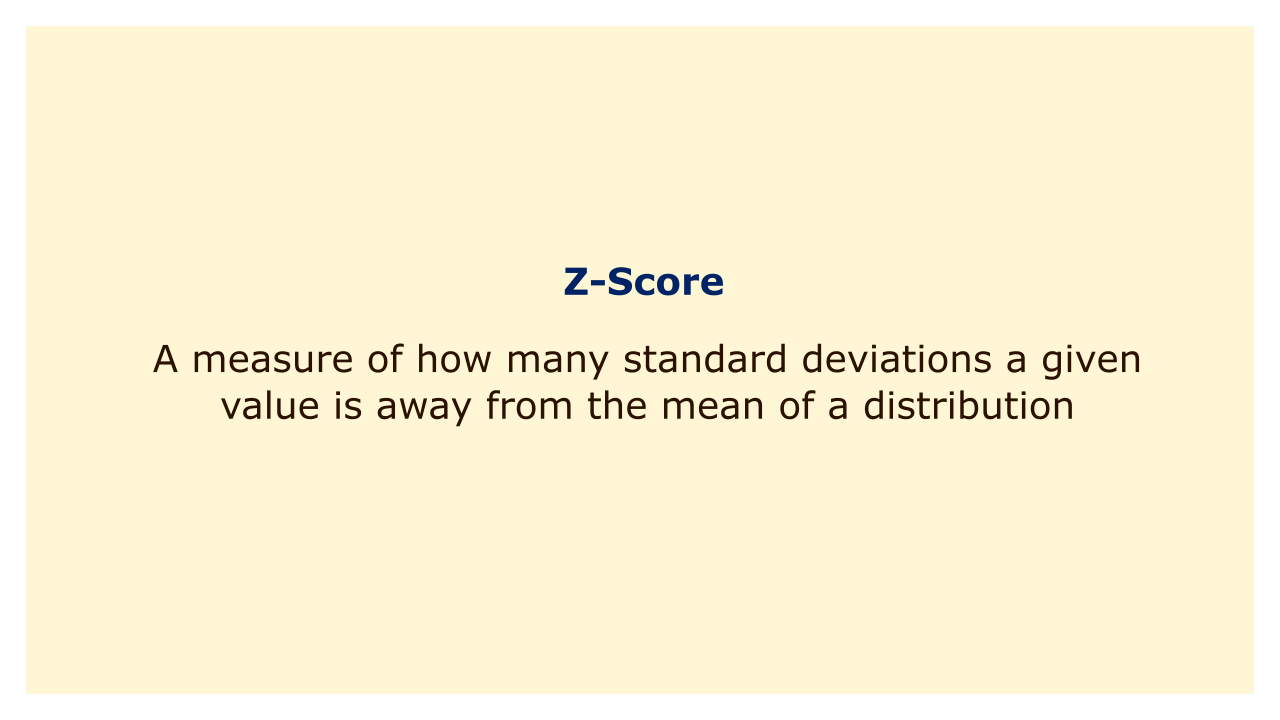# Z-ScoreImage: Moneybestpal.com

### A z-score, often called a standard score, is a measurement of how much a given number deviates from the distribution's mean.

By deducting the mean from the value and dividing by the standard deviation, it is determined. When a distribution's mean is 50 and its standard deviation is 10, for instance, a value of 70 has a z-score of (70 - 50) / 10 = 2, which is considered to be low. So, 70 represents a two-standard deviation increase over the mean.

Z-scores are helpful for contrasting data from various scales or distributions. You can convert two students' test results to z-scores and then compare them to see whether one is higher or lower in relation to each distribution, for instance, if the students took two different tests with different means and standard deviations. Z-scores can also be used to spot outliers, or values that are disproportionately high or low in comparison to the rest of the data. Any value with a z-score larger than 3 or lower than -3 is typically regarded as an anomaly.

Z-scores can also be used to "standardize" a distribution, or change it into a normal distribution with a mean and standard deviation of 0 and 1, respectively. This can facilitate the use of statistical techniques and tests that rely on the assumption of normalcy, such as confidence intervals and hypothesis testing. A distribution can be standardized by simply converting each value to the matching z-score. A value of 120, for instance, has a z-score of (120 - 100) / 20 = 1 and a standardized value of 1, for a distribution with a mean of 100 and a standard deviation of 20, for instance.
Tags